Physics
Fox

### Summary

• A voltmeter is used to measure the potential difference across any electrical component.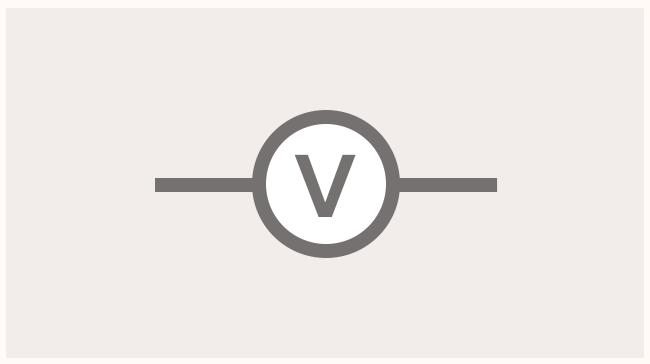• This is found by connecting a voltmeter in parallel with the component: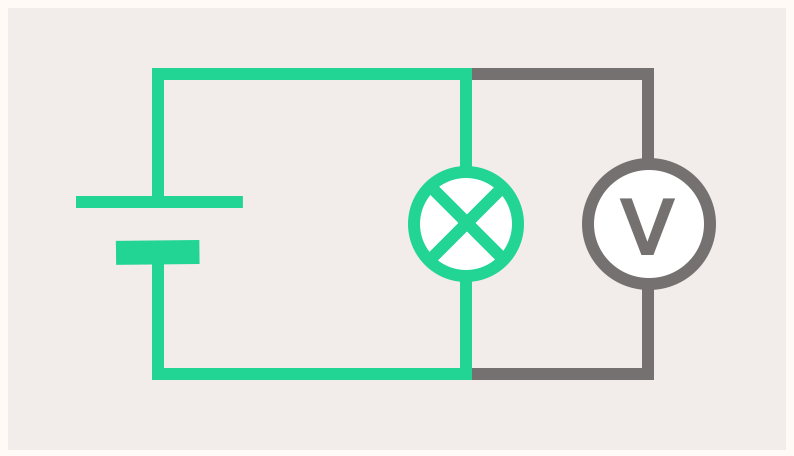A voltmeter measures the voltage across any electrical component. Here's what one might look like in a school lab: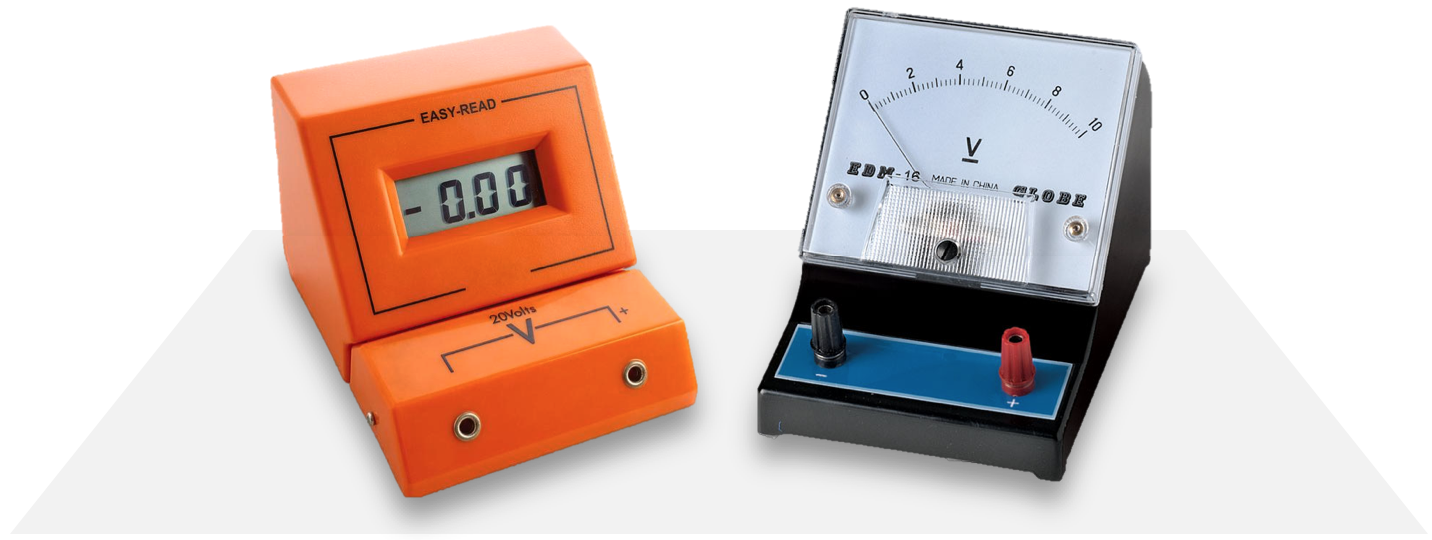Left: A digital voltmeter.
Right: An analogue voltmeter.
Notice how they look pretty similar to ammeters! So if you're using one, pay attention to whether it has an A or V on it.

This is the symbol for a voltmeter, which we'll use on circuit diagrams:So how do we use a voltmeter? It's easy, all we need to do is connect it in parallel with the electrical component we're interested in: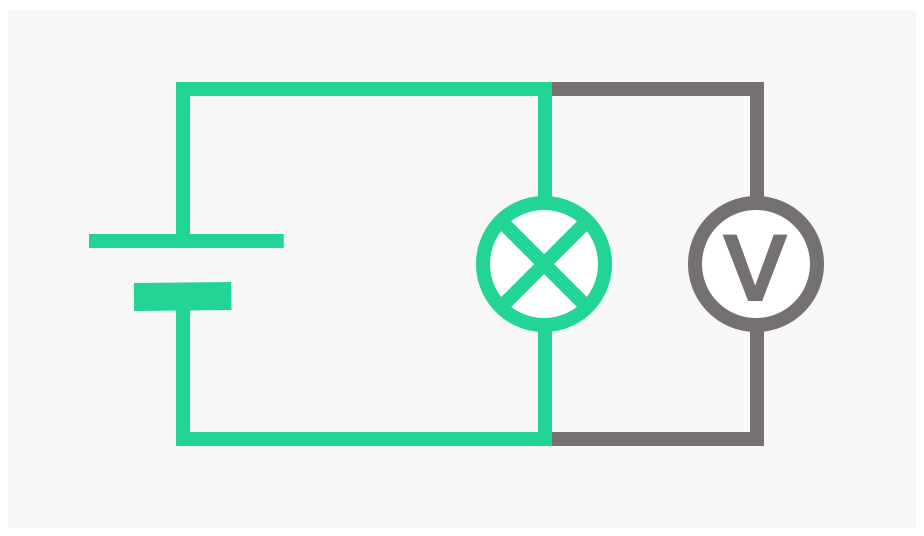So in a complicated circuit like the one below, to measure the potential difference across bulb B all we need to do is 'hug' a voltmeter around it: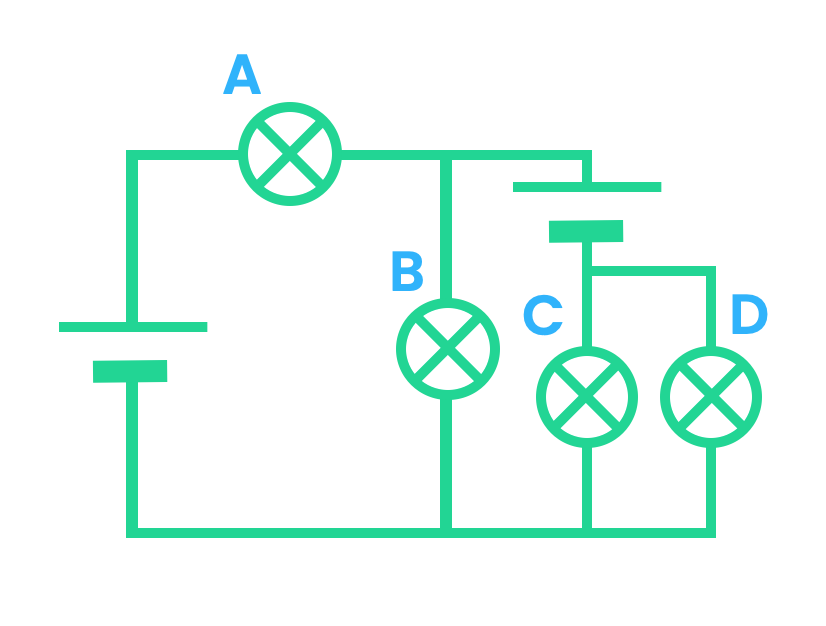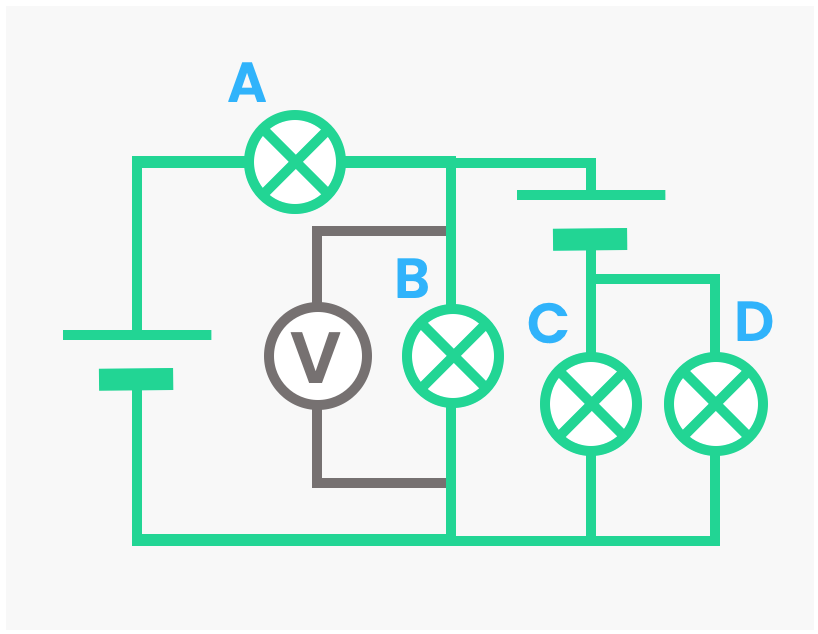In a lab, using a voltmeter is a bit easier than using an ammeter. This is because it's easy to hug a voltmeter around any component we want. To use an ammeter we need to take the circuit apart slightly to put the ammeter in (and then take the circuit apart again to remove it).

A voltmeter doesn't actually measure the voltage across other components — it measures the voltage across itself!

So how does it seem to measure the voltage of other components? Remember: All components that are in parallel always have the same potential difference. Therefore a voltmeter's p.d. is the same as the p.d. of anything it is in parallel with. This is why we have to connect it in parallel!

Outside of a school physics lab, ammeters and voltmeters aren't separate devices; they're combined into one device called a multimeter.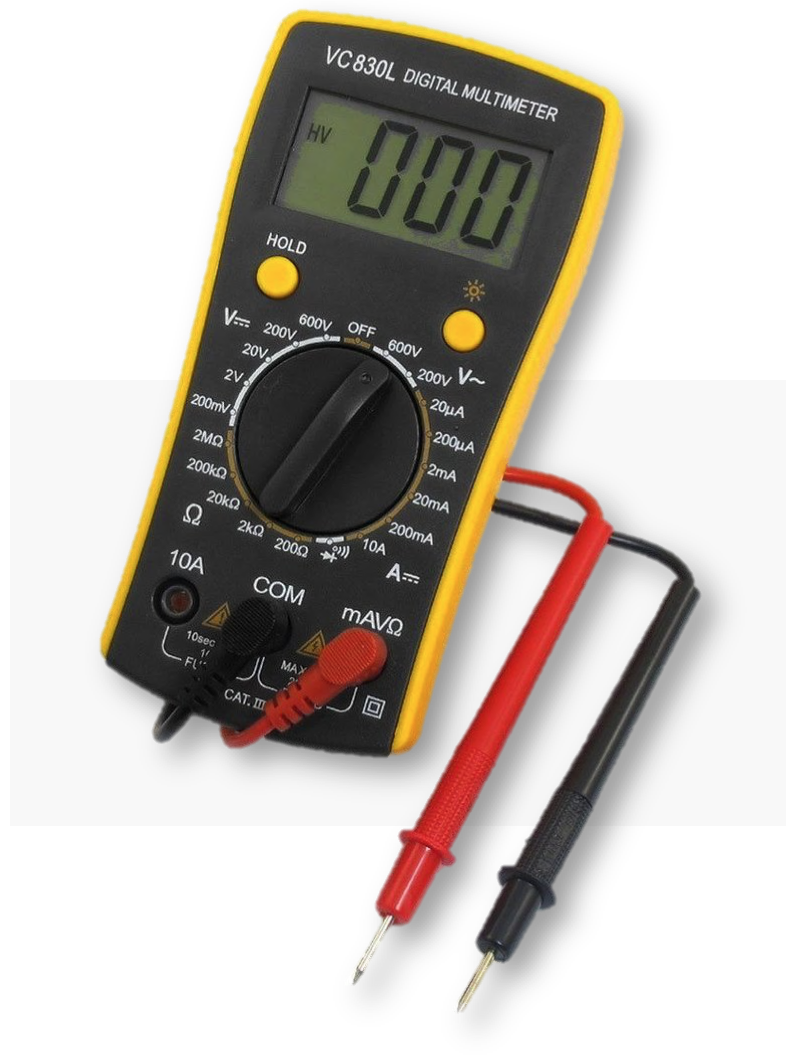A multimeter is the Swiss Army knife of electricity; you can chose whether to use it in ammeter-mode or voltmeter-mode, and how you use each of these is exactly the same as how you use an individual ammeter or voltmeter (like how using Swiss Army knife in knife-mode or scissor-mode works exactly how an individual knife or pair of scissors would normally work).

Multimeters can also measure a property of electrical components called resistance. We'll learn about resistance another time.

Quiz

### Congratulations!

7 of 7 questions completed

+ ⭐️ collected.

• You can change your display name at any time.
• at least 1 letter
• at least 1 number
• at least 8 characters